• ### Browse All Lessons

##### Assign Lesson

Help Teaching subscribers can assign lessons to their students to review online!

 Tweet# Newton’s Laws of Motion

This lesson aligns with Next Generation Science Standards (NGSS) PS2.A

Introduction
A motion is a phenomenon in which an object or body changes its position regarding time. To describe and understand the motion of an object, we must know the term “force” and how it plays a significant role in motion. For instance, we need force to move an object that is at rest, we use force to stop a moving body, we exert force to lift objects, to walk on the road, etc. Thus, force plays an important role in describing the motion of an object. Newton was the first scientist who presented the three laws of motion to describe the scientific relation between force and motion. In this article, we will learn about these three laws of motion.

Newton’s Laws of Motion
Isaac Newton presented three laws of motion to describe the motion of massive bodies and how they interact with each other. These three statements of Newton about motion help us understand how object behave when at rest, when moving, and when any external force act upon them. Force is an important factor in explaining Newton’s laws of motion. The term force is briefly explained below.

Force
Force refers to a push or pulls on an object. It is an external agent that is capable of changing the state of the body, either at rest or moving, e.g., pulling a door. Force is a vector quantity. There is a scientific relation between the forces acting on a body and the motion of the body.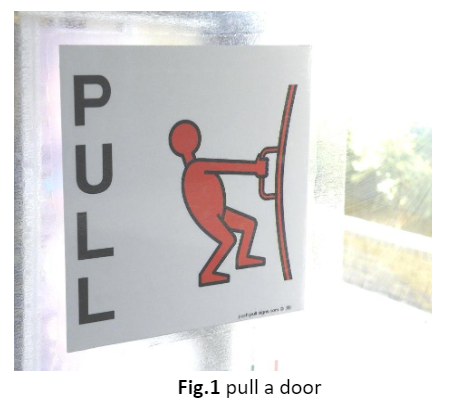First Law of Motion
Newton’s first law of motion implies objects that cannot stop, move or change direction all on their own. They require some force from outside to bring such a change. All objects resist changes in their state of motion until an unbalanced force does not act upon them.

The tendency to resist changes in their state is known as inertia. In other words, inertia is a property of a body that resists any change in its motion, including a change in direction. Therefore, newton’s first law of motion is also known as the law of inertia.

Newton’s first law is stated as “an object stays at rest, and an object in motion remains in motion at constant speed until and unless a net external force acts on it.”

If all the external forces cancel each other, the resulting net force acting on a body is zero. If there is no net force acting on a body, then the object will maintain a constant velocity. However, if any additional force is applied, the velocity will change because of the force.

Newton’s Second Law of Motion
Newton’s second law describes how massive bodies behave when an external force is exerted on them. It is defined as the force acting on the object that is equal to the product of its mass and acceleration.

This law states that “the acceleration of an object that is produced by a net force is directly proportional to the magnitude of a net force and inversely proportional to the mass of the object.”

The acceleration is produced in the same direction as the net force. This law is a quantitative description of the changes that a force can produce in the motion of the object. Mathematically, it can be expressed as;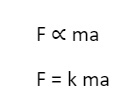Where F is the force, k is the constant of proportionality, m is the mass of the object, and a is the acceleration.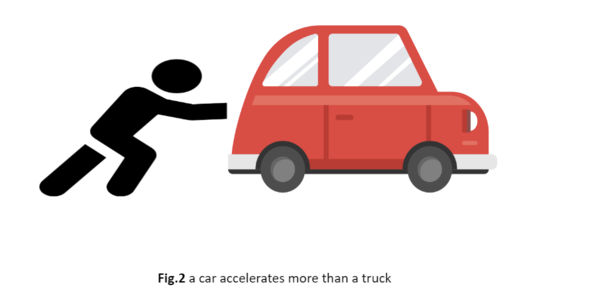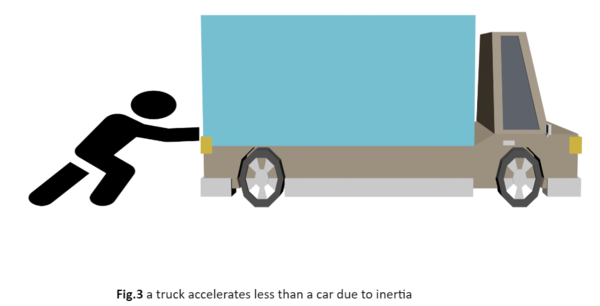For example, when an equal magnitude of the force is applied to a car and a truck, it produces acceleration in both. By comparing the acceleration, it is easy to notice that after pushing a car and a truck with the same magnitude, the car accelerates more than the truck. This is due to inertia, as the mass of the car is less than the mass of the truck.

Newton’s Third Law of Motion
This law states “There is always a reaction to every action that is equal in magnitude and opposite in direction.”

When two bodies come close to each other and interact, they exert forces on each other that are equal in magnitude and opposite in direction. Newton’s third law of motion can be observed with the help of an example, a person swimming in the forward direction pushes the water in the backward direction with his hands. The force applied by a person on water acts as an action force, and as the reaction force, the water causes a person to swim in the forward direction. Both forces are acting together simultaneously, the person’s body and the water.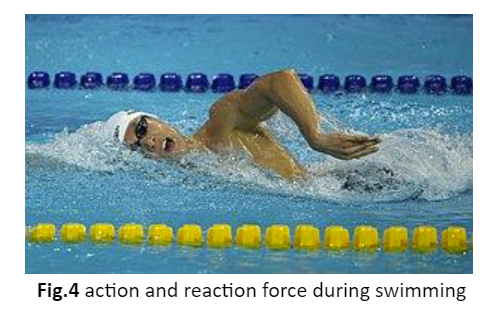Summary
• Newton’s first law of motion describes it as “a body maintains its state either at rest or in motion until an external force acts on it.”
• The second law of motion states as “the force acting on the object is equal to the product of its mass and acceleration.”
• Newton’s third law is defined as “to every action, there is an equal and opposite reaction”.

Related Worksheets: